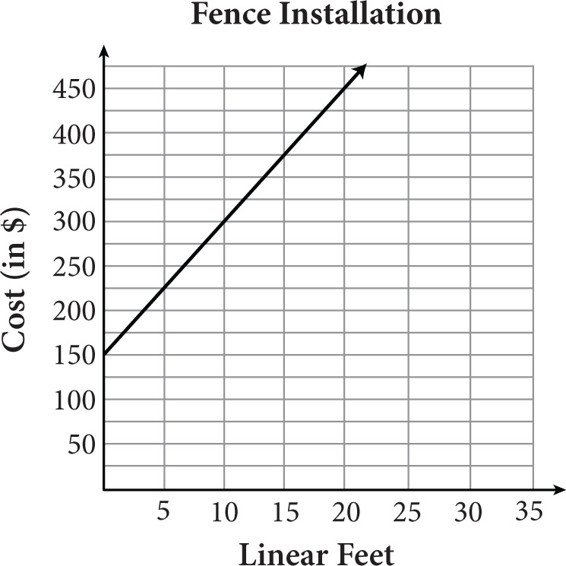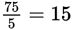# SAT Math Multiple Choice Question 241: Answer and Explanation

### Test Information

Question: 241

1.The graph shows the cost of installing a vinyl privacy fence. The company charges a flat installation fee plus a cost per linear foot of fencing. Based on the graph, how much does one linear foot of this particular vinyl fence cost?

• A. \$5
• B. \$15
• C. \$75
• D. \$150

Getting to the Answer: The cost of one linear foot is the same as the unit rate (the cost per linear foot), which is represented by the slope of the line. Use the grid-lines and the axis labels to count the rise and the run from the y-intercept of the line (0, 150) to the next point that hits an intersection of two grid-lines. Pay careful attention to how the grid-lines are marked (by 5s on the x-axis and by 25s on the y-axis). The line rises 75 units and runs 5 units, so the slope isdollars per linear foot of fence. Note that you could also use the slope formula and two points from the graph to find the unit rate.